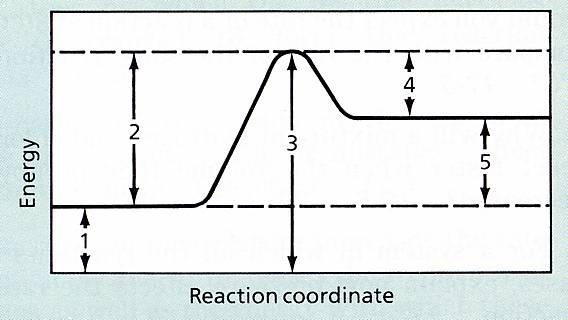# Activation Energy and the Activation Complex

 Page 4/4 Date 23.04.2018 Size 404.21 Kb. #45986
1   2   3   4

### Demonstration 1:

Demonstration 1:

Background: The wax of a candle burns readily in the presence of oxygen.

• Place a candle on the demonstration table and ask students questions like:

• Why is the candle, in the presence of oxygen, not reacting?

• How can we get this reaction to occur?

• What is the function of the match in causing the reaction to occur?

Demonstration 2:

- Create a way to demonstrate activation energies: a suggestion would be rolling a ball up a hill, very similar to the diagram under the potential energy section in these notes.

### Sample Questions

1. Define the terms ‘activation energy’ and ‘activated complex’.

2. Differentiate between activated complex and transition state.

3. What is a potential energy diagram? What does it show?

4. The potential energy diagram shown below is for the hypothetical reaction C A + B.1. Is the reaction exothermic or endothermic?

2. Select the number on the diagram that indicates the

1. activation energy for the forward reaction

(ii) activation energy for the reverse reaction

(iii) potential energy of the reactant

(iv) potential energy of the activated complex

5. In the hypothetical reaction, X + Y Z, the enthalpy of the forward reaction is

H = -36 KJ/mol. the activation energy for the forward reaction is 73 KJ/mol

1. Draw a potential energy level diagram for this reaction. Be sure to label all the

necessary parts.

1. What is the activation energy for the reverse reaction?